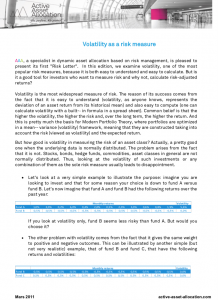# Risk Letter | Volatility as a risk measure

Volatility is the most widespread measure of risk. The reason of its success comes from the fact that it is easy to understand (volatility, as anyone knows, represents the deviation of an asset return from its historical mean) and also easy to compute (one can calculate volatility with a built-­in formula in a spread sheet). Common belief is that the higher the volatility, the higher the risk and, over the long term, the higher the return.

And this is pretty much the basis for Modern Portfolio Theory, where portfolios are optimized in a mean-­variance (volatility) framework, meaning that they are constructed taking into account the risk (viewed as volatility) and the expected return…

The Risk Letter is available in English below :